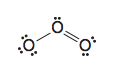# Problem: Ozone (O3) is found in the upper atmosphere where it absorbs highly energetic ultraviolet (UV) radiation and thereby provides the surface of Earth with a protective screen (cf. Section 10.11E). One possible resonance structure for ozone is the following: (a) Assign any necessary formal charges to the atoms in this structure.  (b) Write another equivalent resonance structure for ozone (c) What do these resonance structures predict about the relative lengths of the two oxygen–oxygen bonds of ozone? (d) In the structure above, and the one you have written, assume an angular shape for the ozone molecule. Is this shape consistent with VSEPR theory? Explain your answer

###### FREE Expert Solution
90% (386 ratings)
###### Problem Details

Ozone (O3) is found in the upper atmosphere where it absorbs highly energetic ultraviolet (UV) radiation and thereby provides the surface of Earth with a protective screen (cf. Section 10.11E). One possible resonance structure for ozone is the following:

(a) Assign any necessary formal charges to the atoms in this structure.

(b) Write another equivalent resonance structure for ozone

(c) What do these resonance structures predict about the relative lengths of the two oxygen–oxygen bonds of ozone?

(d) In the structure above, and the one you have written, assume an angular shape for the ozone molecule. Is this shape consistent with VSEPR theory? Explain your answerWhat scientific concept do you need to know in order to solve this problem?

Our tutors have indicated that to solve this problem you will need to apply the Cumulative General Concepts concept. If you need more Cumulative General Concepts practice, you can also practice Cumulative General Concepts practice problems.

What is the difficulty of this problem?

Our tutors rated the difficulty ofOzone (O3) is found in the upper atmosphere where it absorbs...as high difficulty.

How long does this problem take to solve?

Our expert Organic tutor, Jonathan took 2 minutes and 37 seconds to solve this problem. You can follow their steps in the video explanation above.

What professor is this problem relevant for?

Based on our data, we think this problem is relevant for Professor Pollet's class at GT.

What textbook is this problem found in?

Our data indicates that this problem or a close variation was asked in Organic Chemistry - Solomons 10th Edition. You can also practice Organic Chemistry - Solomons 10th Edition practice problems.